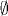# SETS AND SEQUENCES

Sets are the most basic concepts in measure theory as well as in mathematics. In fact, set theory is a foundation of mathematics (Moschovakis, 2006). The algebra of sets develops the fundamental properties of set operations and relations. In this chapter, we shall introduce basic concepts about sets and some set operations such as union, intersection, and complementation. We will also introduce some set relations such as De Morgan’s laws.

# 1.1 Basic Concepts and Facts

Definition 1.1 (Set, Subset, and Empty Set). A set is a collection of objects, which are called elements. A set B is said to be a subset of a set A, written as BA, if the elements of B are also elements of A. A set A is called an empty set, denoted by, if A contains no elements.

Definition 1.2 (Countable Set). A set A is said to be countable if either A contains a finite number of elements or every element of A appears in an infinite sequence x1, x2,…. A set A is said to be uncountable if it is not countable.

Definition 1.3 (Equality of Sets). Two sets A and B are said to be equal, written as A = B, if AB and BA.

Definition 1.4 (Union, Intersection, and Complement of Sets). Let A and B be two subsets of a set S. The union of A and B is defined asThe intersection of A and B is defined ...

Get Measure, Probability, and Mathematical Finance: A Problem-Oriented Approach now with the O’Reilly learning platform.

O’Reilly members experience live online training, plus books, videos, and digital content from nearly 200 publishers.